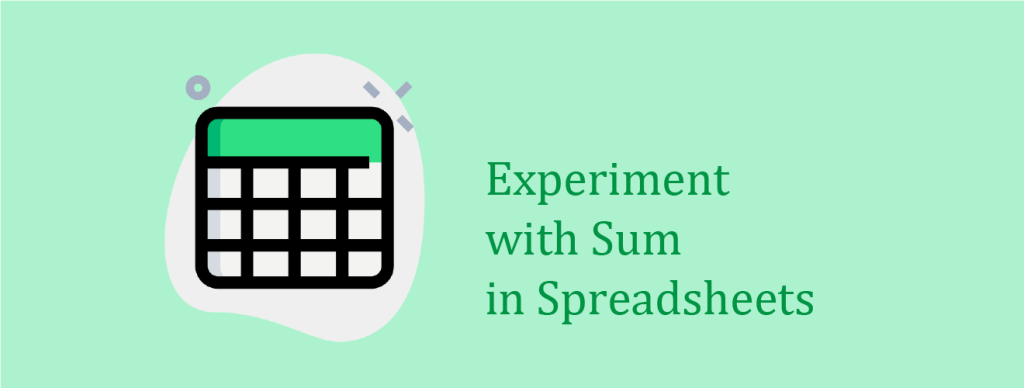# Experiment with Sum in Spreadsheets

There is more than one way to write a formula. In a spreadsheet, there are many ways to write an addition formula. Try them! Which way do you like the best?

You have surveyed your friends to find which color of candy they like best. You would like to know the total number of people you surveyed. Using Google Sheets or Microsoft Excel, open a blank spreadsheet and enter the following information. Make sure you enter the first color – blue – in cell A4.

Survey your friends or use this sample data.

Sum with the plus sign between each cell:

1. Select a blank cell.
2. Type the formula =B4+B5+B6+B7+B8+B9+B10+B11+B12

Sum with a comma between each cell:

1. Select a blank cell.
2. Type the formula =SUM(B4,B5,B6,B7,B8,B9,B10,B11,B12)

Sum with a cell range:

1. Select a blank cell.
2. Type the formula =SUM(B4:B12)

Sum by naming a cell range:

1. Select the cells with the votes (B4:B12).
2. From the Data menu, select Named ranges.
3. Replace NamedRange1 with votes. Click Done.
4. Select a blank cell.